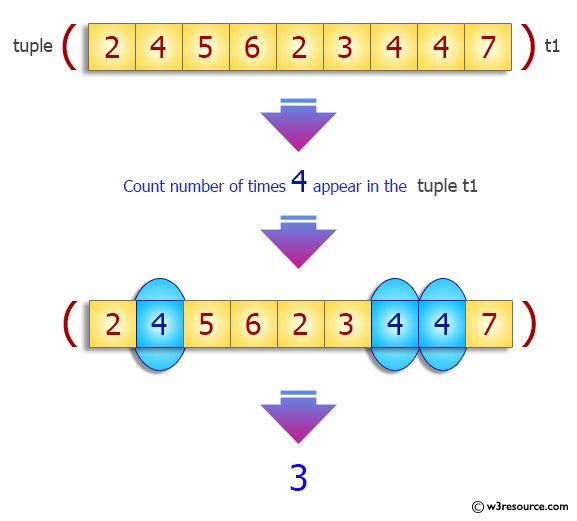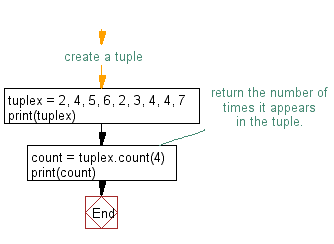﻿ Python: Repeated items of a tuple - w3resource# Python Exercise: Repeated items of a tuple

## Python tuple: Exercise-9 with Solution

Write a Python program to find the repeated items of a tuple.

Sample Solution:-

Python Code:

``````#create a tuple
tuplex = 2, 4, 5, 6, 2, 3, 4, 4, 7
print(tuplex)
#return the number of times it appears in the tuple.
count = tuplex.count(4)
print(count)
```
```

Sample Output:

```(2, 4, 5, 6, 2, 3, 4, 4, 7)
3
```

Pictorial Presentation:Flowchart:## Visualize Python code execution:

The following tool visualize what the computer is doing step-by-step as it executes the said program:

Python Code Editor:

Have another way to solve this solution? Contribute your code (and comments) through Disqus.

What is the difficulty level of this exercise?

Test your Programming skills with w3resource's quiz.

﻿

## Python: Tips of the Day

Decapitalizes the first letter of a string:

Example:

```def tips_decapitalize(s, upper_rest=False):
return s[:1].lower() + (s[1:].upper() if upper_rest else s[1:])
print(tips_decapitalize('PythonTips'))
print(tips_decapitalize('PythonTips', True))
```

Output:

```pythonTips
pYTHONTIPS
```